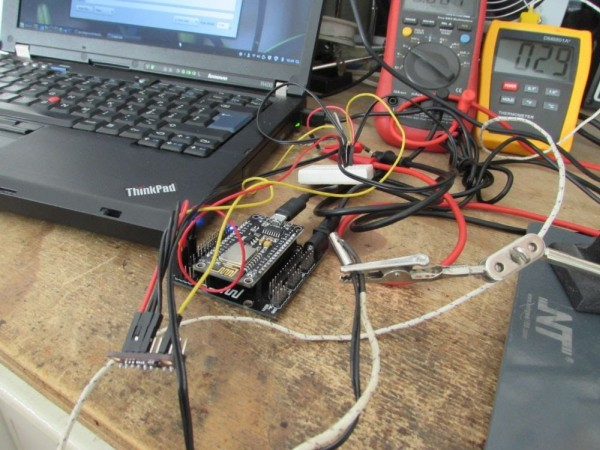esp8266/Arduino NTC library

A thermistor is a type of negative coefficient resistor whose resistance is dependent on temperature, more so than in standard resistors. The resistance of a NTC Negative Temperature Coefficient thermistor (https://en.wikipedia.org/wiki/Thermistor) decreases as temperature rises. The Steinhart-Hart Thermistor Equation or the Beta Model Equation can be used to correlate the thermistor resistance to temperature to which the sensor is exposed.

The library implements the Steinhart-Hart Thermistor Equation or the Beta Model Equation to convert adc value read from analog input to temperature.
It output temperatures in Celsius or Fahrenheit.

The ESP8266 comes with a 10bit ADC, 0 to 1V, one can use the ESP8266 ADC for this library.
Suppose you are going to the internal ADC, and that NTC is going to be used between -20 and +100 degree Celsius. The NTC resistance by datasheet at -20 degree is 100k, and at 100 degree 100. To evaluate the pullup resistor you have to consider that the Voltage output at the ADC pin will be
Vout = Vpullup*(Rntc/Rntc+Rpullup)
We have to consider the max Vout, that comes from the max resistance given by the NTC
Voutmax = Vpullup*Rntcmax/(Rntcmax+Rpullup)
We have to chose a Rpullup such that Voutmax < 1V, so:
Rpullup = Vpullup*Rntcmax/Voutmax – Rntcmax
Subsitute with the sample NTC we are considering:
Rpullup = 3.3*100000/1 – 100000 = 230000.
A 220k resistor would do the job.

In the example i propose here I am using a ADS1015 12 bit analog to digital comparator, that is much more accurate than the internal ESP8266 ADC.
I am using the Adafruit ADS1X15 library for this IC, on top of that library I’ve build an helper to just convert the ADC raw value that comes from the ADS1x15 to a resistance or voltage value.

READ  Arduino Online Thermometer ProjectOnce we’ve acquired the NTC resistance we can use the Steinhart-Hart Thermistor Equation or the Beta Model Equation to convert the resistance value to temperature.

A digital iir filter is implemented to remove unwanted reading errors.

This library was developed on Atom+PlatformIO, compiled esp8266/Arduino.

Code

Read More: esp8266/Arduino NTC library

This Post / Project can also be found using search terms:

• esp8266 library
• production7s1